# Python Program to Check Vowel or Consonant

In this article, we've created some programs in Python to check whether a character entered by user at run-time is a vowel or not. Here are the list of programs:

• Check Vowel or Not using if-else
• Using list
• Using function
• Using class

Before creating these programs, let's first understand about vowels in brief.

### What is a Vowel ?

If the pronunciation of a character pronounced by humans, when the breath flows out through mouth without being blocked by teeth, lips or tongue. Then the character is called as vowel.

### List of Vowels

Basically there are 5 vowels that are:

• a, e, i, o, u
• A, E, I, O, U

Note - Sometime, y can also be called as a vowel. But all programs created here, based on five vowels.

## Check Vowel or Consonant using if-else

To check whether the input character is a vowel or consonant in Python, you have to ask from user to enter a character, then check and print the message as shown in the program given below. The question is, write a Python program to check vowel or consonant. Here is its answer:

```print("Enter the Character: ")
c = input()

if c=='a' or c=='e' or c=='i' or c=='o' or c=='u':
print("\nIt is a Vowel")
elif c=='A' or c=='E' or c=='I' or c=='O' or c=='U':
print("\nIt is a Vowel")
else:
print("\nIt is a Consonant")```

Here is the initial output produced by this Python program: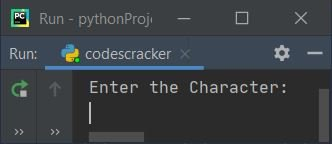Now supply the input say e as character and press `ENTER` key to check whether it is a vowel or a consonant, and print the message as shown in the snapshot given below: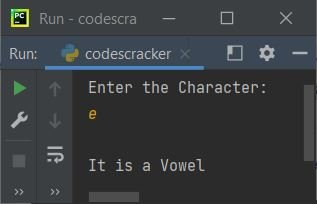#### Modified Version of Previous Program

This is the modified version of previous program. This program uses end to skip printing of an automatic newline using print(). The len() method is used to find the length of string. And \" is used to print " on output.

```print(end="Enter a Character: ")
c = input()

size = len(c)
if size>1:
print("\nInvalid Input!")
else:
if (c>='a' and c<='z') or (c>='A' and c<='Z'):
if c=='a' or c=='e' or c=='i' or c=='o' or c=='u':
print("\n\"" +c+ "\" is a Vowel")
elif c=='A' or c=='E' or c=='I' or c=='O' or c=='U':
print("\n\"" +c+ "\" is a Vowel")
else:
print("\n\"" +c+ "\" is a Consonant")
else:
print("\n\"" +c+ "\" is neither a Vowel nor a Consonant")```

Here is its sample run with user input, C: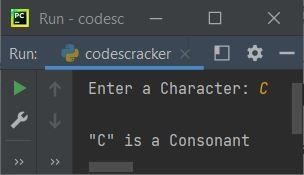Here is another sample run with user input, 4: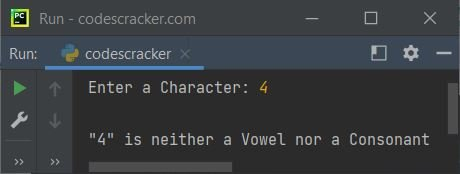And here is the last sample run with user input 4r (two characters (string) input):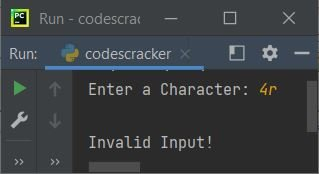## Check Vowel or Consonant using List

This program is created using list. That is, we've created a list named vowels that contains all vowels. Therefore, through this list, we've checked the entered character by user, whether the character present in the list using in or not.

```print(end="Enter a Character: ")
c = input()

size = len(c)
if size>1:
print("\nInvalid Input!")
else:
if (c>='a' and c<='z') or (c>='A' and c<='Z'):
vowels = ['a', 'e', 'i', 'o', 'u', 'A', 'E', 'I', 'O', 'U']
if c in vowels:
print("\n\"" +c+ "\" is a Vowel")
else:
print("\n\"" +c+ "\" is a Consonant")
else:
print("\n\"" +c+ "\" is neither a Vowel nor a Consonant")```

This program produces exactly same output as of previous program.

## Check Vowel or Consonant using Function

This program uses a user-defined function named checkVowel() to check whether a character entered by user is a vowel or consonant.

```def checkVowel(x):
if (c>='a' and c<='z') or (c>='A' and c<='Z'):
vowels = ['a', 'e', 'i', 'o', 'u', 'A', 'E', 'I', 'O', 'U']
if c in vowels:
return 1
else:
return 2

print(end="Enter a Character: ")
c = input()

size = len(c)
if size>1:
print("\nInvalid Input!")
else:
chk = checkVowel(c)
if chk==1:
print("\nVowel")
elif chk==2:
print("\nConsonant")
else:
print("\nNeither Vowel nor Consonant")```

Here is its sample run with user input, U: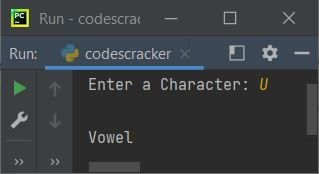The function checkVowel() returns 1 if the character passed as its argument is a vowel, otherwise returns 2 in case of consonant.

## Check Vowel or Consonant using Class

This is the last program of this article, created using class, an object-oriented feature of Python. This program does the same job as of previous program.

```class CodesCracker:
def checkVowel(self, x):
if (c>='a' and c<='z') or (c>='A' and c<='Z'):
vowels = ['a', 'e', 'i', 'o', 'u', 'A', 'E', 'I', 'O', 'U']
if c in vowels:
return 1
else:
return 2

print(end="Enter a Character: ")
c = input()

size = len(c)
if size>1:
print("\nInvalid Input!")
else:
ob = CodesCracker()
chk = ob.checkVowel(c)
if chk==1:
print("\nVowel")
elif chk==2:
print("\nConsonant")
else:
print("\nNeither Vowel nor Consonant")```

This program produces similar output as of previous program. An object ob of class CodesCracker is created to access its member function named checkVowel() through dot (.) operator

#### Same Program in Other Languages

Python Online Test

« Previous Program Next Program »